# Why vignetting factors should be removed when calculating Relative Illumination and MTF

• 651 views

Userlevel 6+2
• Zemax Staff
• 108 replies

Sometimes users are confused why the 'Remove Vignetting Factors' option in the analysis Relative Illumination is by default checked on. Also some may wonder why the operand RELI always removes the vignetting factors. In this post, let's discuss and explain why it's usually not a good idea to use vignetting factor when evaluating the system's RI or MTF.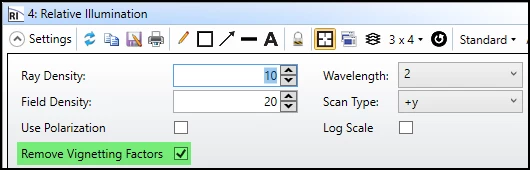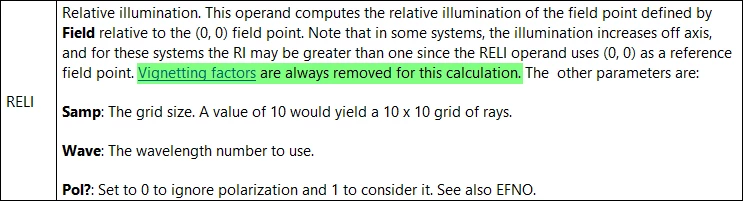To explain, let's open an example and examine what happens if we turn on and off the vignetting factors.

1. Open '\Documents\Zemax\Samples\Sequential\Objectives\Double Gauss 28 degree field.zmx'

2. Run 'Design Lockdown' to fix the sysetm before we start evaluations.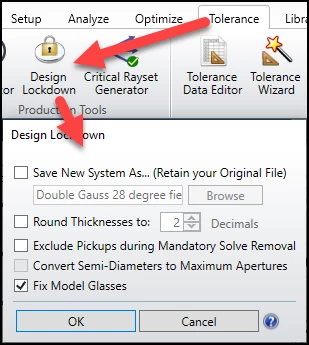3. Let's check Footprint Diagram on STOP (surface 6) with Wavelength = 2 and Field = 2. For now, as expected, the beam can fulfill the STOP.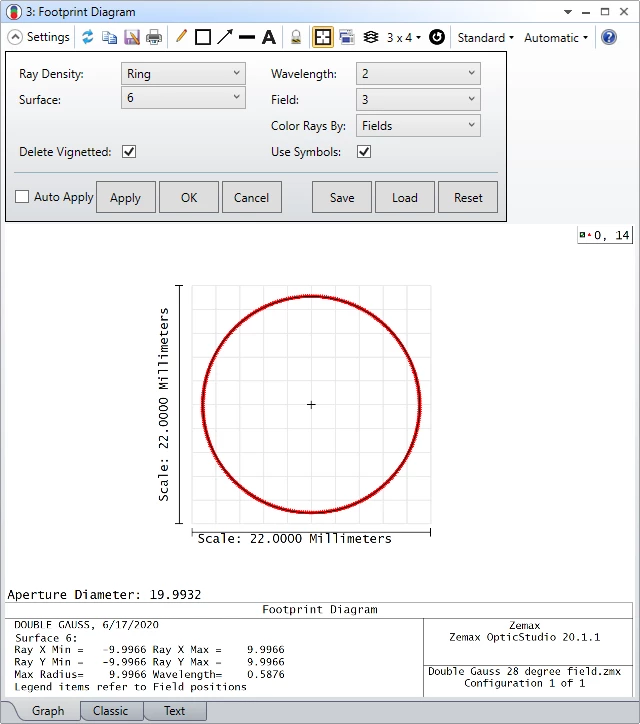4. Now let's make the final surface's aperture smaller as below.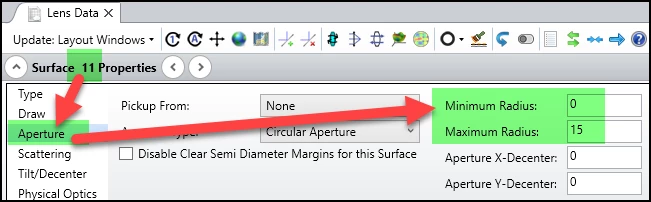5. As expected, the Footprint Diagram changes as below.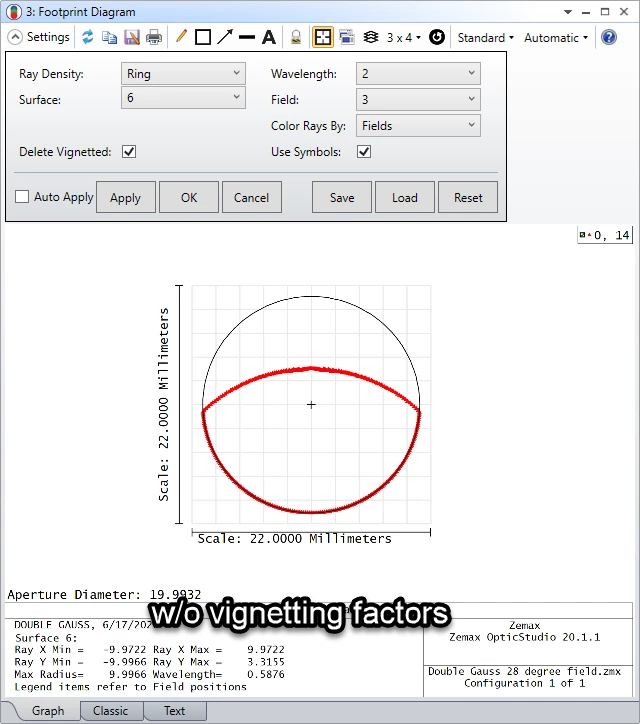6. Here is how the smaller aperture changes Layout and Relative Illumination.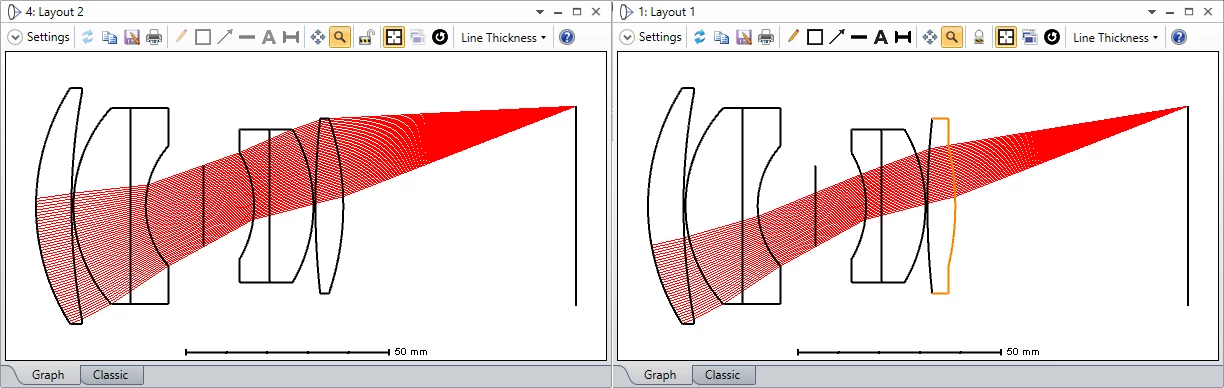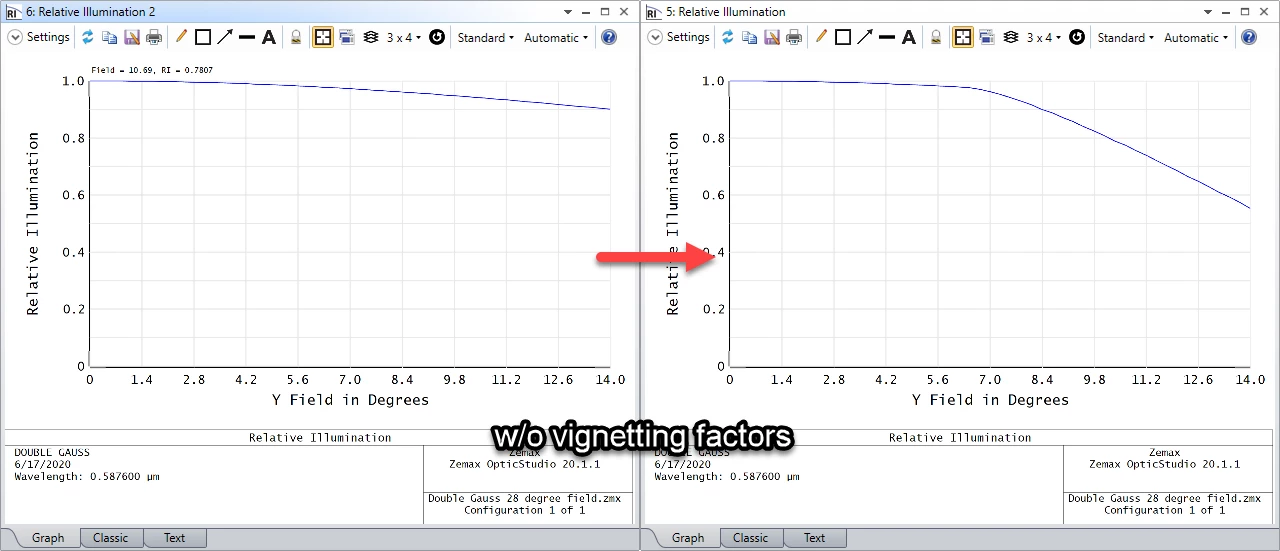7. Now let's set the vignetting and see how it changes the system.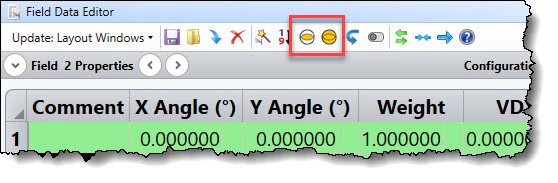8. It can be seen the vignetting factors can actually 'over shrink' the beam in Footprint Diagram. From this view point, it's clear the result with considering vignetting factors is actually incorrect becuase some part of the beam is not considered. (green area is larger than red area)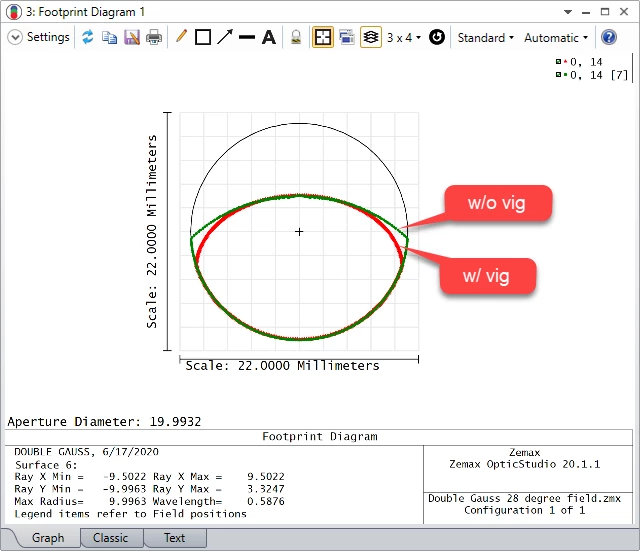9. In Relative Illumination, the result is also different. Note it doesn't always cahnge like below. It could be the RI is higher when considering vignetting factors.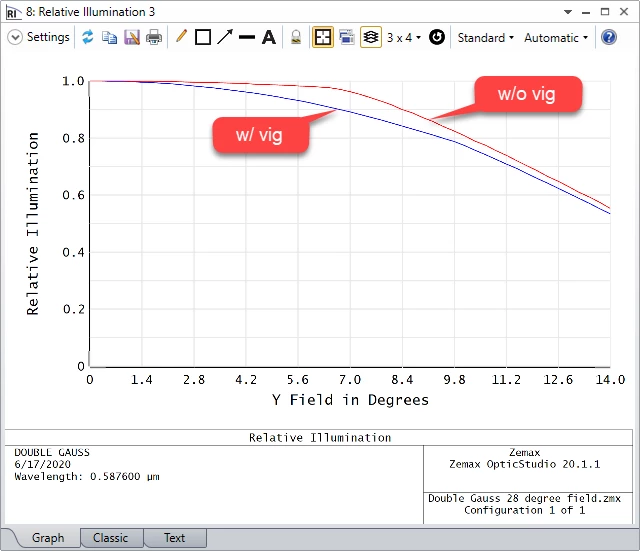10. In below, the result of FFT MTF is also shown for reference. The red lines consider the vignetting factors and the green lines do not consider.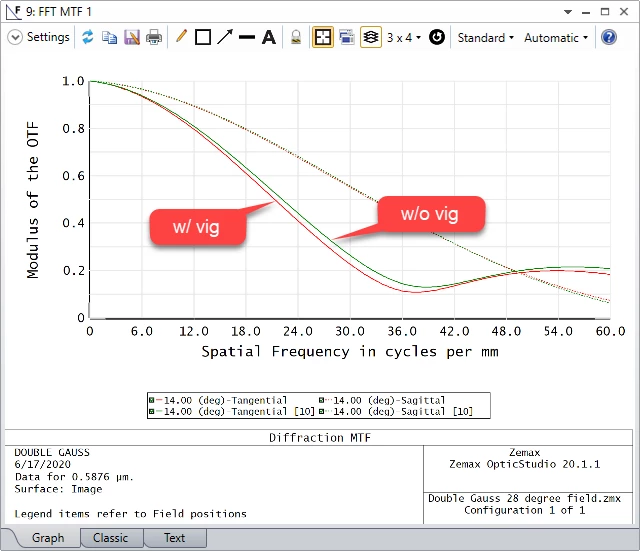To understand how vignetting factors affect the beam shape & size, the following is two suggested article to read for further understanding!